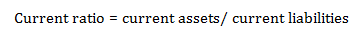# Current ratio – Meaning & formula

Current ratio is one of the liquidity ratios, shows the relationship between current assets and current liabilities of a firm. Current ratio indicates a firm’s commitment to meet its short-term debt obligations.

If the value of this ratio is greater than 1, then it indicates that the company is in position to pay its current liabilities by liquidating its current assets. A value of lesser than 1 indicates that the company can not pay its current liabilities by liquidating all of its current assets.

This ratio is also known as ‘working capital ratio’.

### Formula for the calculation of current ratio:

This ratio is calculated by dividing the amount of current assets by current liabilities as shown below#### Example:

Let’s suppose XYZ ltd reports total current assets of INR 80,000 at the end of financial year 2015 – 16 and current liability of INR 75,000.

Working capital ratio (for the financial year 2016 – 17) = 80,000 / 75,000 = 1.07

This shows that company is in position to pay its current liabilities.

Posted on Categories Accounting & Finance

## 2 thoughts on “Current ratio – Meaning & formula”

1.Beatrice says:

1.Vikas Yadav says: